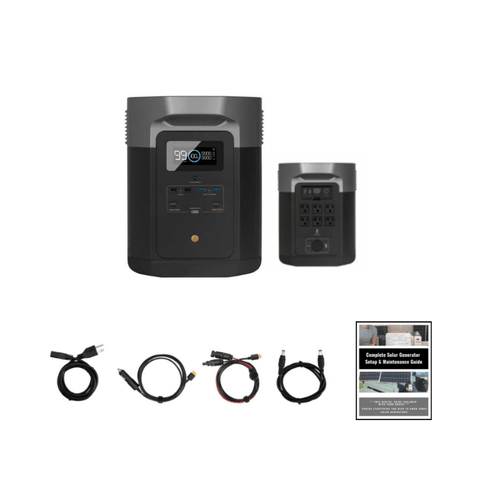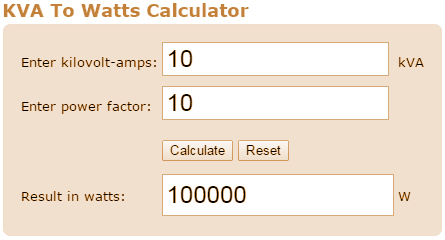Convert KVA to Watts: How Do I Do It? - ShopSolar.com

# Convert kVA to Watts

We’ve created a valuable guide for those looking to convert KVA to Watts. Both of these are units used to measure power, however, they measure power in slightly different ways.

We’ll go over these differences to help you understand their application in a solar power setting.

## What’s a kVA?

kVA refers to a kilo-volt amp, which is 1,000 volt-amps. To find the kVA value of a unit, you multiply the voltage by the amps.

## What’s a Watt?

A watt is the unit used to measure power. It’s the amount of energy something needs to function or the rate at which something uses energy to move.

Smaller power stations have a lower wattage, whereas larger ones have a higher wattage.

If you’re on the lookout for a portable power station, check out our EcoFlow Delta MAX portable power station.## kVA in a Solar Power System

kVA measures the apparent power in an electrical system. This is not to be confused with kilowatts, which measure the true power of an electrical system.

Some units will express the amount of power they hold in kVA, but it’s more useful to know the power in Watts, as this is the measure of true power.

So what do you do if your system is measured in kVA, and you want to know how much power it can hold in Watts?

You calculate it! Below, we’ve included simple steps you can follow to the math.

Remember, converting kVA to watts is only one of the many equations you need to understand in order to properly maintain your solar panel system. Be sure to check out our milliamp-hour to watt calculator to wrap your head around another important conversion.

## kVA to Watts Calculation

The calculation is fairly simple and easy to do. We’ll explain it here, and illustrate how to use it with an example.

The process has two steps. First, we’ll convert the kVA to kW, or kilowatts. We’ll then convert the kW to Watts.

If you’re looking for other conversion charts, check out our guide on how to convert volts to watts.### Calculation

The first formula is as follows:

kW = kVA x PF.

In words, this reads as kilowatts equals kilovolt amps multiplied by the power factor.

But what’s the power factor? Well, remember that we mentioned apparent power and true power earlier? The power factor is the ratio between these two.

The ideal power factor for a solar power system is 1, which indicates that no extra power is needed to operate the system than it generates. 0.9 is, however, a more common power factor for these systems.

We’ll work with that value to illustrate how to use this calculation.

In terms of the kVA value, we’ll work with 1.5 kVA. This is a reasonable amount of power and is quite common. A solar power system like this could power a refrigerator, a few lights, your laptop, and your TV.

So, let’s fill in the values and see what we get.

kW = kVA x PF

kW = 1.5 kVA x 0.9

kW = 1.35

So now we have the kilowatt capacity of the system. How do we turn that into Watts? Easy!One kilowatt is equal to one thousand watts. From this, we can determine the following:

1.35 kilowatts is equal to 1,350 watts.